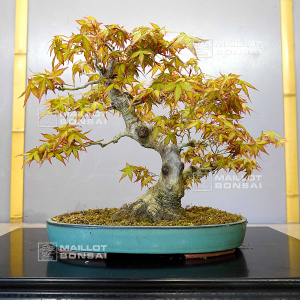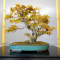##### The Japanese Bonsai specialist
Direct order Contact Help / Services Newsletter# Acer palmatum 17030227

Yama momijiref. : 116271.240,00

Available quantity : 1Order

###### Description

Height of the tree only : 250 mm. Width of the branches : 320 mm. Dimensions of the Japanese enamelled pot : 285*205*40 mm.

This bonsaï comes from seedlings, it is more than 20 years old with its veined formed bark.

Moyogi style, perfectly well ramfied. Beautiful tones in fall.

This subject has thin and natural ramifications. Good conicity of the trunk. Formed nebari, no strong cutting wound. The cutting wounds that are still visible are almost healed under they grey mastic. No wire mark.

It will require 2 years more to finish the ramifications of the trays. Shelf not included. Coming from  Master Murakawa's nursery, Fukuya, Japan.

For collector.

Photographed without its foliage in March 2022.

#bonsai 3.6 #ramifications 3.3 #palmatum 2.8 #cutting 2.7 #formed 2.6 #years 2.5 #this 2.4 #more 2.4 #mini 2.4 #acer 2.4

Formule
(( ROUND((CHAR_LENGTH(b.article_nom)-CHAR_LENGTH(REPLACE(b.article_nom, 'ramifications', '')))/LENGTH('ramifications')) + ROUND((CHAR_LENGTH(b.article_description)-CHAR_LENGTH(REPLACE(b.article_description, 'ramifications', '')))/LENGTH('ramifications')) ) * 3.3) + (( ROUND((CHAR_LENGTH(b.article_nom)-CHAR_LENGTH(REPLACE(b.article_nom, 'palmatum', '')))/LENGTH('palmatum')) + ROUND((CHAR_LENGTH(b.article_description)-CHAR_LENGTH(REPLACE(b.article_description, 'palmatum', '')))/LENGTH('palmatum')) ) * 2.8) + (( ROUND((CHAR_LENGTH(b.article_nom)-CHAR_LENGTH(REPLACE(b.article_nom, 'cutting', '')))/LENGTH('cutting')) + ROUND((CHAR_LENGTH(b.article_description)-CHAR_LENGTH(REPLACE(b.article_description, 'cutting', '')))/LENGTH('cutting')) ) * 2.7) + (( ROUND((CHAR_LENGTH(b.article_nom)-CHAR_LENGTH(REPLACE(b.article_nom, 'formed', '')))/LENGTH('formed')) + ROUND((CHAR_LENGTH(b.article_description)-CHAR_LENGTH(REPLACE(b.article_description, 'formed', '')))/LENGTH('formed')) ) * 2.6) + (( ROUND((CHAR_LENGTH(b.article_nom)-CHAR_LENGTH(REPLACE(b.article_nom, 'years', '')))/LENGTH('years')) + ROUND((CHAR_LENGTH(b.article_description)-CHAR_LENGTH(REPLACE(b.article_description, 'years', '')))/LENGTH('years')) ) * 2.5) + (( ROUND((CHAR_LENGTH(b.article_nom)-CHAR_LENGTH(REPLACE(b.article_nom, 'this', '')))/LENGTH('this')) + ROUND((CHAR_LENGTH(b.article_description)-CHAR_LENGTH(REPLACE(b.article_description, 'this', '')))/LENGTH('this')) ) * 2.4) + (( ROUND((CHAR_LENGTH(b.article_nom)-CHAR_LENGTH(REPLACE(b.article_nom, 'more', '')))/LENGTH('more')) + ROUND((CHAR_LENGTH(b.article_description)-CHAR_LENGTH(REPLACE(b.article_description, 'more', '')))/LENGTH('more')) ) * 2.4) + (( ROUND((CHAR_LENGTH(b.article_nom)-CHAR_LENGTH(REPLACE(b.article_nom, 'acer', '')))/LENGTH('acer')) + ROUND((CHAR_LENGTH(b.article_description)-CHAR_LENGTH(REPLACE(b.article_description, 'acer', '')))/LENGTH('acer')) ) * 2.4) + (( ROUND((CHAR_LENGTH(b.article_nom)-CHAR_LENGTH(REPLACE(b.article_nom, 'photographed', '')))/LENGTH('photographed')) + ROUND((CHAR_LENGTH(b.article_description)-CHAR_LENGTH(REPLACE(b.article_description, 'photographed', '')))/LENGTH('photographed')) ) * 2.2) + (( ROUND((CHAR_LENGTH(b.article_nom)-CHAR_LENGTH(REPLACE(b.article_nom, 'dimensions', '')))/LENGTH('dimensions')) + ROUND((CHAR_LENGTH(b.article_description)-CHAR_LENGTH(REPLACE(b.article_description, 'dimensions', '')))/LENGTH('dimensions')) ) * 2)

## Secure payment## Delivery

Our logistic partners :04 74 55 23 48
Pépinière MAILLOT-BONSAÏ
Le Bois Frazy
01990 RELEVANT - FRANCE
on appointment